본문바로가기

## 검색결과

검색결과 (전체 1건)

### 검색결과제한

열기
• 원문
• 발행년도
-
• 언어
• 원문이용안내
•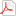모든 이용자
•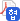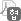협정기관 이용자
•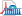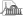국회도서관 방문 이용자
• 원문아이콘이 없는 자료는 국회도서관에 방문하여 책자로만 이용이 가능합니다.

논문명/저자명
A study on adaptive interference management techniques in LTE-A based enterprise femtocell networks = LTE-Advanced 기반의 기업형 펨토셀 네트워크에서 적응적인 간섭 관리 기술에 관한 연구 / Sang-Joon Lee
발행사항
서울 : 고려대학교 대학원, 2013.2
청구기호
TD 004 -13-215
형태사항
xvi, 126 p. ; 26 cm
자료실
서고(열람신청 후 1층 논문실)
제어번호
KDMT1201304635
주기사항
학위논문(박사) -- 고려대학교 대학원, Dept. of Computer Science, 2013.2. 지도교수: 조충호
원문

목차보기더보기

Title Page

ABSTRACT

Contents

LIST OF ABBREBIATIONS 17

CHAPTER 1. INTRODUCTION 20

1.1. Introduction 20

1.2. Motivation 22

1.3. Problem Statement 23

1.4. Thesis Contribution 24

1.5. Organization of the Thesis 24

CHAPTER 2. BACKGROUND AND LITERATURE REVIEW 26

2.1. Introduction 26

2.2. Standardization 27

2.3. Evolution before LTE 29

2.4.1. Long Term Evolution 32

2.4.2. System Structure 39

2.4.3. Physical Channel 41

2.5. Femtocell Technology 49

2.5.1. Femtocell Concept 49

2.5.2. The Need for Femtocell 51

2.5.3. Interference Mitigation in Femtocell 53

2.6. Inter-Cell Interference Management in Heterogeneous Networks 55

2.6.1. Inter-Cell Interference Management 55

2.6.2. Almost Blank Subframes 62

CHAPTER 3. GENERAL INTERFERENCE COORDINATION FOR ENTERPRISE FEMTOCELL NETWORKS 66

3.1. Introduction 66

3.2. System Models 66

3.2.1. Simulation Environment 66

3.2.2. System Assumption 67

3.2.3. Channel Model 68

3.3. Performance analysis of mUE/fUE by position of EFN 70

3.3.1. Simulation Results 70

3.4. Summary 77

CHAPTER 4. NETWORK ARCHITECTURE FOR ENTERPRISE FEMTOCELL NETWORKS 78

4.1. Introduction 78

4.2. System Model 79

4.2.1. Simulation Environment 79

4.2.2. Simulation Assumption 79

4.2.3. Simulation parameters 80

4.2.4. Performance Indicator 81

4.2.5. Femtocell and ABS 81

4.3. Performance Analysis of EFN Structure for EFN 82

4.3.1. Proposed Architecture using Interface b/w Macrocell and EFN 82

4.3.2. Proposed System Structures 84

4.3.3. Results 86

4.4. Performance Analysis of EFN using SS for Control Channel 90

4.4.1. Three Schemes using ABS and SS to avoid Interference 91

4.4.2. Additional Schemes using ABS and SS to avoid Interference 92

4.4.3. Results 94

4.5. Summary 98

CHAPTER 5. INTERFERENCE COORDINATION USING ABS FOR ENTERPRISE FEMTOCELL NETWORKS 99

5.1. Introduction 99

5.2. System Model 100

5.2.1. Simulation Environment 100

5.2.2. System Assumption 102

5.2.3. Performance Indicators 103

5.3. Interference Mitigation Schemes for Data Channel 106

5.3.1. Almost blank subframe Resource Control(ARC) 107

5.3.2. Femtocell Resource Control(FRC) 108

5.3.3. ABS & Femtocell Resource Control Scheme(AFRC) 111

5.3.4. Simulation Results 112

5.4. Interference Coordination using ABS for Data Channel 120

5.4.1. Adaptive ABS Resource Control(A-ARC) 120

5.4.2. Adaptive ABS & Femtocell Resource Control Scheme(A-AFRC) 124

5.4.3. Simulation Results 126

5.5. Summary 134

CHAPTER 6. CONCLUSION AND FUTURE RESEARCH 136

6.1. Conclusion 136

REFERENCE 138

Table 2.1. Channel bandwidth 41

Table 3.1. System environment summary 67

Table 4.1. Simulation parameters 80

Table 5.1. The parameter of (5.1) 103

Table 5.2. The parameter of (5.2) 104

Table 5.3. The parameter of (5.3) 104

Table 5.4. The MCS table of (5.3) 105

Table 5.5. The parameter of (5.4) 105

Table 5.6. The parameter of FRC algorithm 108

Table 5.7. The pseudocode of FRC algorithm 109

Table 5.8. The parameter of A-ARC algorithm 121

Table 5.9. The pseudocode of A-ARC algorithm 122

Table 5.10. Result Summary 135

Figure 2.1. Timeline of the Mobile Communication standards 28

Figure 2.2. 3GPP members 29

Figure 2.3. Evolution of Cellular Communication 31

Figure 2.4. Overall E-UTRAN Architecture 33

Figure 2.5. Overall E-UTRAN Architecture with deployed HeNB GW 37

Figure 2.6. Frame structure of LTE system using FDD 39

Figure 2.7. Channel group of LTE system 40

Figure 2.8. Logical and physical channel of LTE system 42

Figure 2.9. Allocated position of PBCH 44

Figure 2.10. Allocated position of PDCCH 45

Figure 2.11. Allocated position of PCFICH 46

Figure 2.12. Allocated position of PHICH 47

Figure 2.13. Control channel mapping 48

Figure 2.14. Comparison of cell sizes of different technologies 51

Figure 2.15. Projected Mobile Data Traffic Growth 56

Figure 2.16. Frame structure for heterogeneous networks with eICIC(Almost blank subframes are applied for TDM-based control and data ICIC) 63

Figure 2.17. e-ICIC solutions: time shifting 64

Figure 3.1. Simulation environment 67

Figure 3.2. 3-sector mBS anatenna 69

Figure 3.3. The fUE outage probability by WL and distance b/w mBS and EFN 71

Figure 3.4. The SIR of fUE in EFN(Dist. b/w mBS : 100m) 72

Figure 3.5. The SIR of fUE in EFN(Dist. b/w mBS : 300m) 73

Figure 3.6. The SIR of mUE in EFN(Dist. b/w mBS : 100m) 74

Figure 3.7. The SIR of mUE in EFN(Dist. b/w mBS : 300m) 75

Figure 3.8. The mUE/fUE outage probability by distance b/w mBS and EFN 76

Figure 3.9. The outage probability zone of 5% in EFN 77

Figure 4.1. Simulation Topology 79

Figure 4.2. The subframe of femtocell that used ABS 82

Figure 4.3. LTE/LTE-A Architecture(Conventional) 83

Figure 4.4. LTE/LTE-A Architecture for EFN(Proposed) 84

Figure 4.5. System structure by interface(Scheme 1) 85

Figure 4.6. System structure by receiver of fBS(Scheme 2) 85

Figure 4.7. System structure by signal analysis of fUE(Scheme 3) 86

Figure 4.8. Effective SINR CDF of PCFICH by system structures 87

Figure 4.9. Effective SINR CDF of PDCCH by system structures 88

Figure 4.10. Effective SINR CDF of PHICH by system structures 89

Figure 4.11. Example of interference b/w macrocell and picocell 90

Figure 4.12. Example of interference avoidance methods using ABS and SS 92

Figure 4.13. The additional interference problem by symbol shifting 93

Figure 4.14. Additional Schemes using ABS and SS to avoid Interference 94

Figure 4.15. Effective SINR CDF of PCFICH by ABS and SS 95

Figure 4.16. Effective SINR CDF of PDCCH by ABS and SS 96

Figure 4.17. Effective SINR CDF of PHICH by ABS and SS 97

Figure 5.1. Simulation environment of sub-chapter 5.3 100

Figure 5.2. Simulation environment of sub-chapter 5.4 101

Figure 5.3. Example of interference on subframe b/w macrocell and femtocell 106

Figure 5.4. Example of ABS allocation by ARC 107

Figure 5.5. The flow chart of Femtocell Resource Control(FRC) algorithm 110

Figure 5.6. Example of resource allocation by AFRC scheme 111

Figure 5.7. The SINR CDF of mBS subframe 112

Figure 5.8. The SINR CDF of fBS subframe 113

Figure 5.9. The throughput of mBS subframe 115

Figure 5.10. The throughput of fBS subframe 115

Figure 5.11. The outage probability of mBS subframe 117

Figure 5.12. The outage probability of fBS subframe 118

Figure 5.13. UEs's Information reception of EFNS through interface 120

Figure 5.14. The flow chart of Adaptive ABS Resource Control(A-ARC) algorithm 123

Figure 5.15. Example of ABS allocation by A-ARC algorithm of A-AFRC scheme 124

Figure 5.16. Example of resource allocation by FRC algorithm of A-AFRC scheme 125

Figure 5.17. The variation of number of ABSs 126

Figure 5.18. The SINR CDF of mBS subframe 128

Figure 5.19. The SINR CDF of fBS subframe 128

Figure 5.20. The throughput of mBS subframe 130

Figure 5.21. The throughput of fBS subframe 130

Figure 5.22. The outage probability of mBS subframe 132

Figure 5.23. The outage probability of fBS subframe 133

## 권호기사보기

권호기사 목록 테이블로 기사명, 저자명, 페이지, 원문, 기사목차 순으로 되어있습니다.
기사명 저자명 페이지 원문 기사목차
연속간행물 팝업 열기 연속간행물 팝업 열기